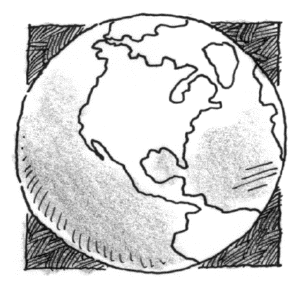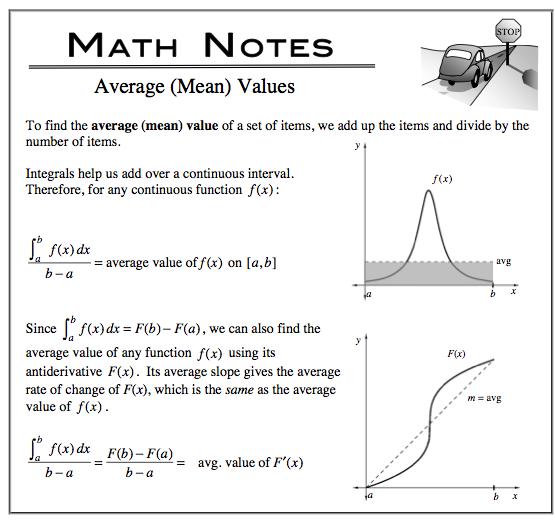### Home > CALC > Chapter 7 > Lesson 7.1.3 > Problem7-35

7-35.In order to plan for the future water supply, the average population of the world is needed. If the world population in 2000 was about $6.1$ billion people, determine the average population of the earth during the following $30$ years. Assume the population, in billions, $t$ years from 2000 is projected to be:

$P(t) = 6.1e^{0.014t}$

You are asked to determine the average value of $P(t)$, given the $P(t)$ function itself. Which variation of Average Value will you use?